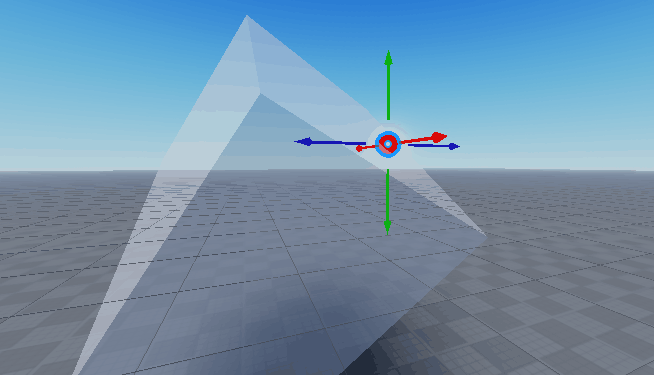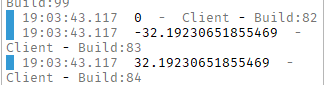# Check if position is in part

YES THE SIMPLE METHODS HAVE BEEN ATTEMPED (e.g. GetTouchingParts(), ZonePlus module, comparing individual X,Y,Z components etc)

How would I ACCURATELY check if a position is within the interior of a block? As mentioned above, the common methods found on DevForum do not work.

Cheers

1 Like
``````function isPointInPart(point, part)
local p = part.CFrame:PointToObjectSpace(point)
local s = part.Size/2
return p.X >= -s.X and p.X <= s.x and p.Y >= -s.Y ... etc
end
``````
2 Likes

This code does not work. I mentioned:

There just may be an issue with my experience, I’ll keep experimenting.

It works fine:It first transforms the point to the part’s local space, so it’s as if the part is centered at 0,0,0 and has no rotation.

That’s weird. I’ll try it again and if it doesn’t work I’ll let you know.

1 Like
``````function checkValidity(pos)
local part = game.Workspace.BuildingStuff.BuildingArea
local p = part.CFrame:PointToObjectSpace(pos)
local s = part.Size/2
print(p.X)
print(-s.X)
print(s.X)
return (p.X >= -s.X and p.X <= s.X) and (p.Y >= -s.Y and p.Y <= s.Y) and (p.Z >= -s.Z and p.Z <= s.Z)
end
``````(always returns true even if out of the area)

Don’t know what to tell you, it works fine for me. Can you try inserting this model (in a blank place, don’t insert untrusted models in your project places) and see if you can get it to work?

test.rbxm (3.9 KB)

Alternatively, here’s the script

``````
function isPointInPart(point, part)
local p = part.CFrame:PointToObjectSpace(point)
local s = part.Size/2
return (p.X >= -s.X and p.X <= s.X) and (p.Y >= -s.Y and p.Y <= s.Y) and (p.Z >= -s.Z and p.Z <= s.Z)
end

while wait() do
local c = isPointInPart(script.Parent.Point.Position, script.Parent.Volume) and BrickColor.Green() or BrickColor.Red()
script.Parent.Point.BrickColor = c
end
``````

And here’s the structure1 Like

Even with your example, it doesn’t work. This baffles me.

1 Like

This is my solution to the problem. It’s a little messy, I guess, but it, so far, has never failed me.

``````local a = workspace.Part1                   -- The part in who's region you want the position to be in.
local b = Vector3.new(30,45,10)        -- The position you want to check. (I just put a random one for an example)
-- The following equations will return you a Vector3Value which describes the distance of position B from the position of part A, while also considering the LookVector, RightVector, UpVector, etc.
local checkZAxis = a.CFrame.LookVector*(b - a.Position)
local checkXAxis = a.CFrame.RightVector*(b - a.Position)
local checkYAxis = a.CFrame.UpVector*(b- a.Position)
-- From here you will have to individually check to see if the part is within each axis.
if math.floor(math.abs(checkXAxis.X + checkXAxis.Z)) < a.Size.X/2 then -- You will have to add both the X and Z axes for the returned checkXAxis Vector3 to get the magnitude. Same goes for the checkZAxis Vector3.
if math.floor(math.abs(checkYAxis.Y)) < a.Size.Y/2 then
if math.floor(math.abs(checkZAxis.X + checkZAxis.Z)) < a.Size.Z/2 then
-- The point is within part A.
end
end
end

``````

Can you save a place with the model inserted where it doesn’t work for you and upload that place file?

Won’t this work?

``````script.Parent.Touched:Connect(function(Hit)
local bool = false
local mag = (script.Parent.Position - Hit.Position).Magnitude
if Hit.Position.X > mag or Hit.Position.Y > mag or Hit.Position.Z > mag then
bool = true
print(bool)
end
end)
``````

The issue is that it DOES work, outside this script. But it doesn’t make sense since nothing in the script is interfering with it. Perhaps the inputs are incorrect? I’ll try some stuff.
Also @Ai_Kyle it appears it has failed me rather than you lol

1 Like

All these solutions offered should work fine. I even tested mine out several times to make sure it worked properly. If none of them are working, then maybe your issue has to do with something else.

2 Likes

I’ll mark this as the solution as it works, but specifically not for my situation to help others find the answer

1 Like

This topic was automatically closed 14 days after the last reply. New replies are no longer allowed.